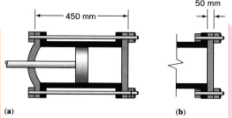Home / Expert Answers / Mechanical Engineering / two-possible-designs-of-a-hydraulic-cylinder-are-being-considered-as-shown-in-figure-a-and-b-on-pa358

# (Solved): Two possible designs of a hydraulic cylinder are being considered as shown in Figure (a) and (b). On ...

Two possible designs of a hydraulic cylinder are being considered as shown in Figure (a) and (b). One involves the use of long bolts and the other uses short bolts of the same diameter. On the basis that the bolts are each required to absorb the same amount of strain energy, decide which of the two designs would be the best.We have an Answer from Expert